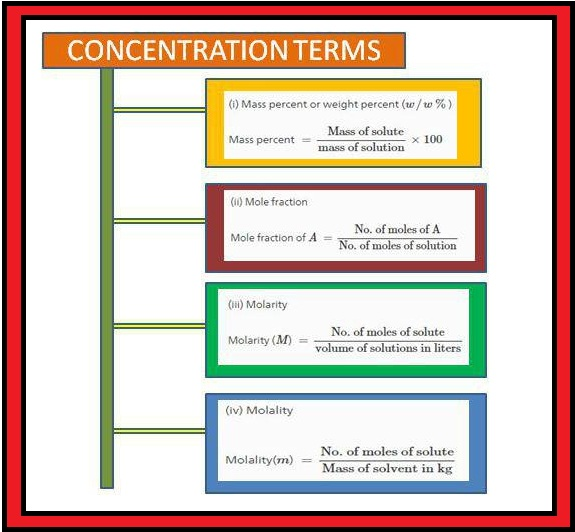Chemistry Quick revision of Basic concepts of chemistry
Click for Only Video

### Nature of matter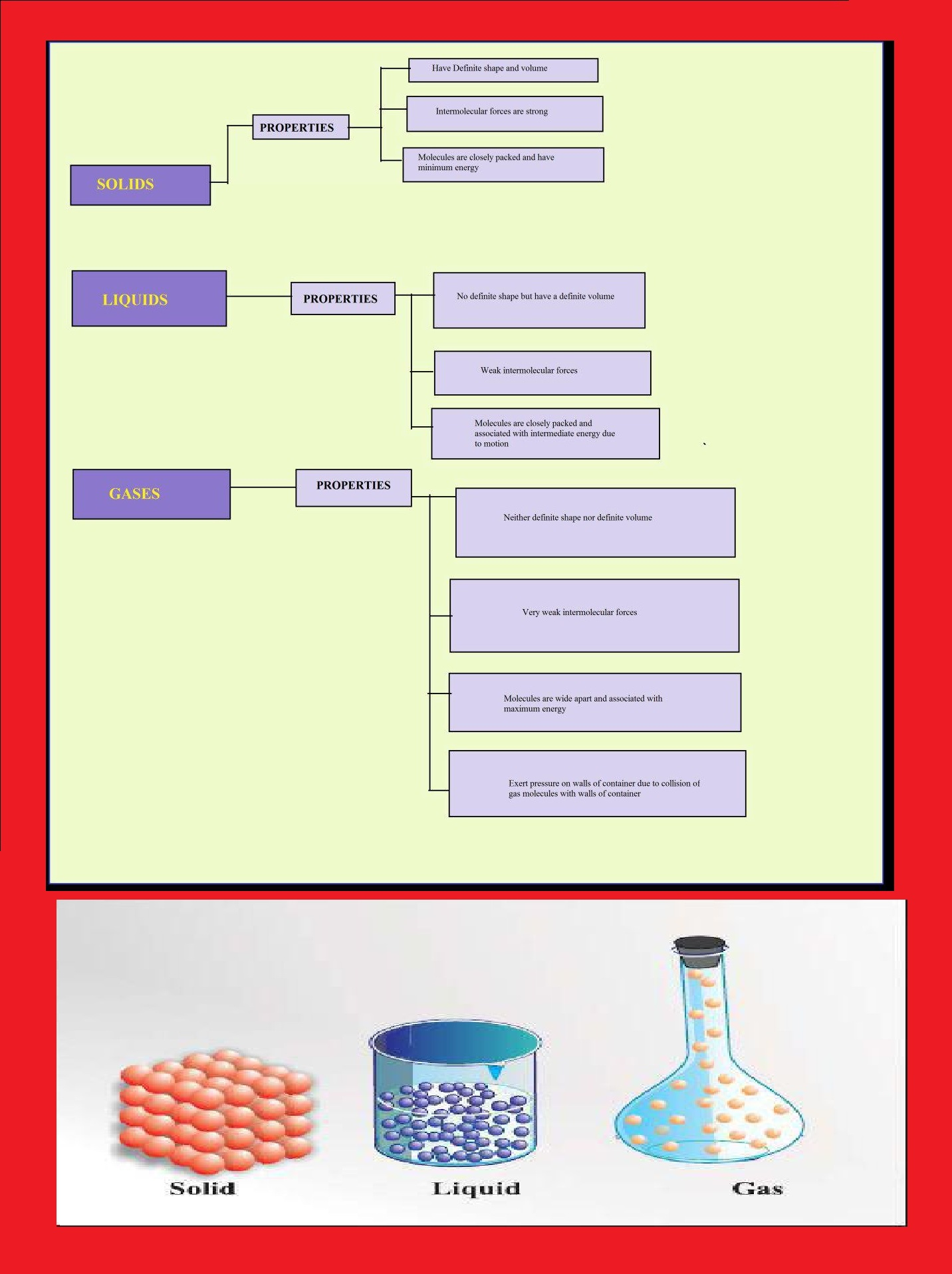### Classification of matter### Properties of matter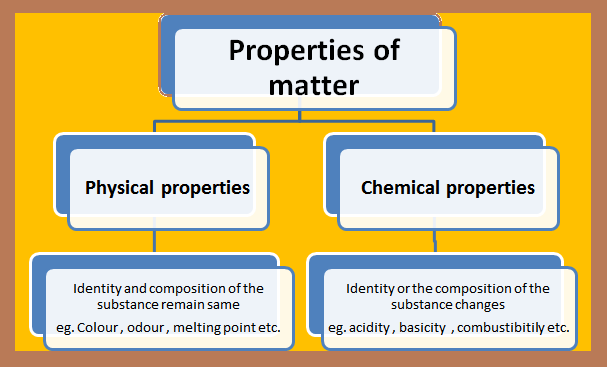### The international system of units (SI)### Quantity used frequently in chemistry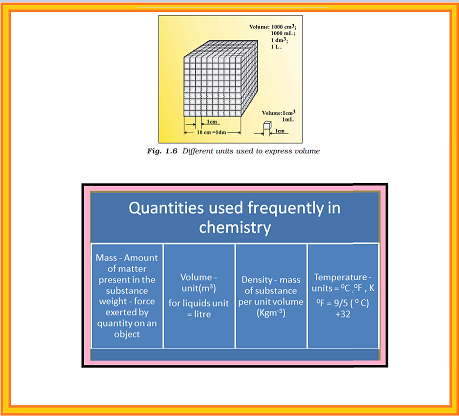### Scientific Notation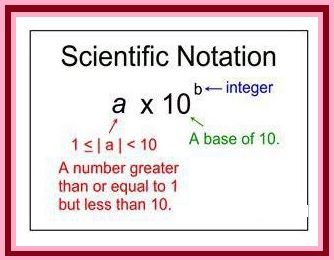### Mathematical operation using scientific notation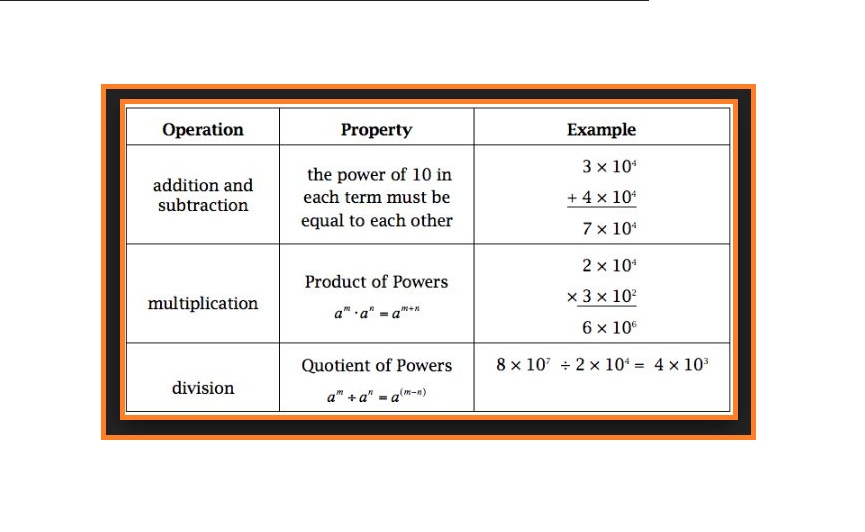### Significant figuresPrecision : It is the closeness of various measurements for the same quantity .

Accuracy : It is the agreement of a particular value to the true value of the result.

### Rules for determining the number of significant figures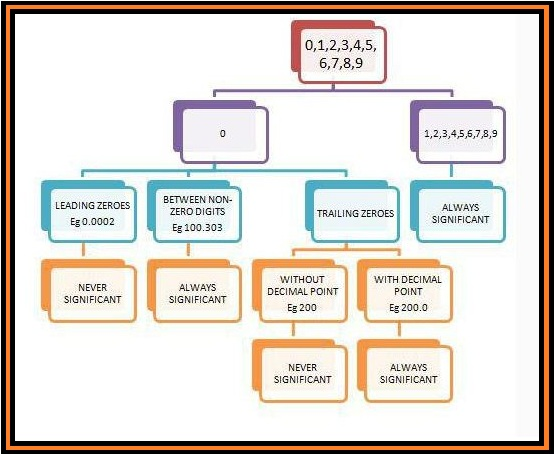### Mathematical operations of significant figures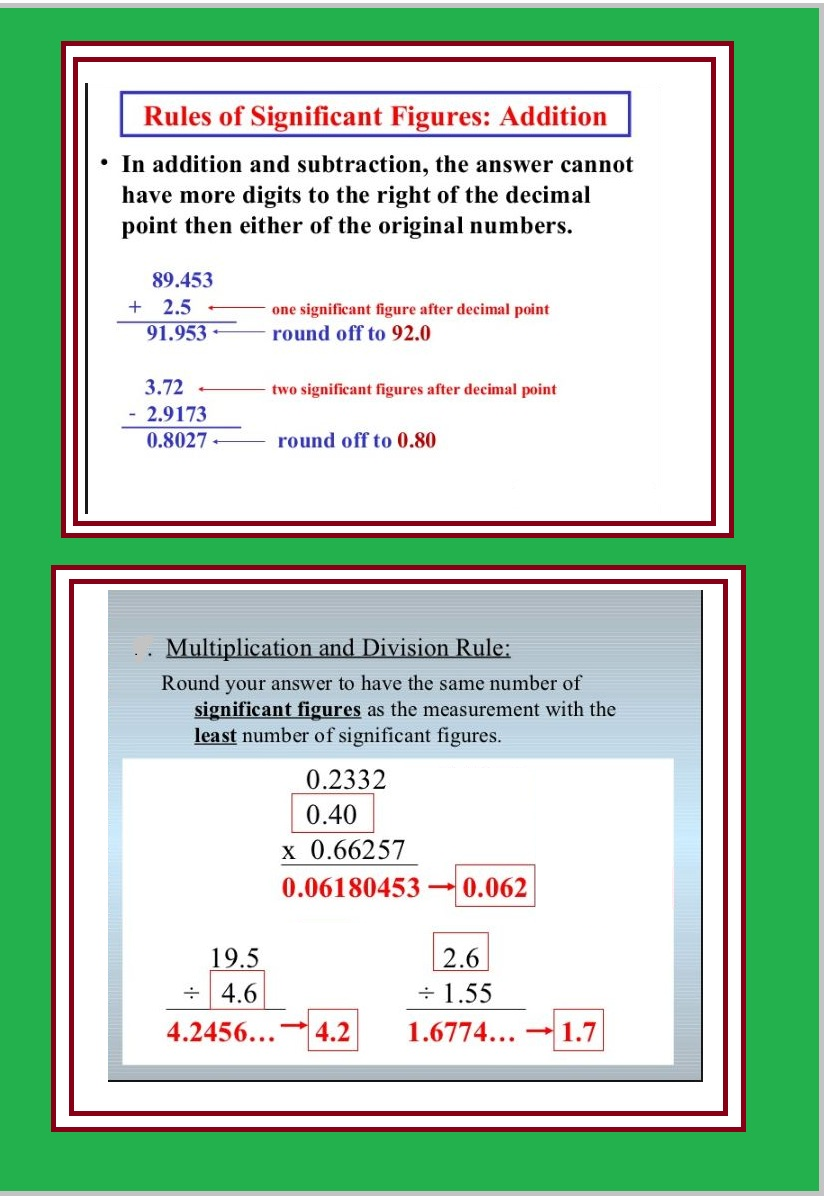### Rules for rounding off the numbers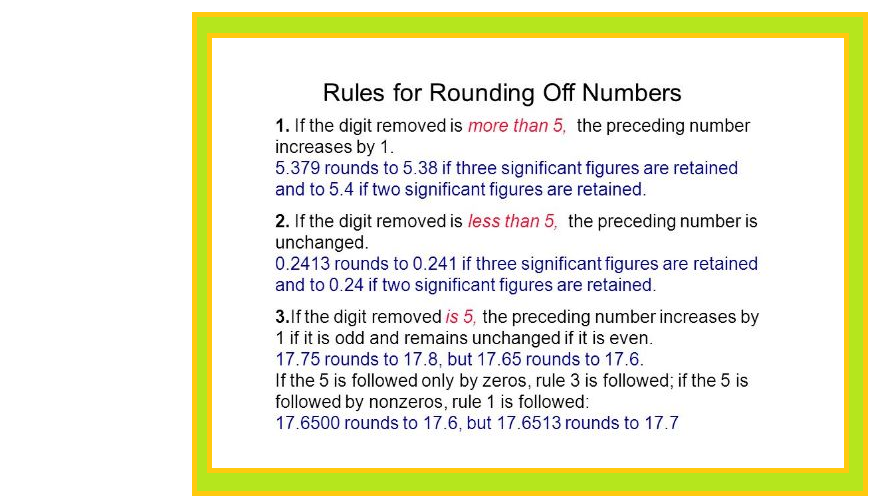### Dimensional analysis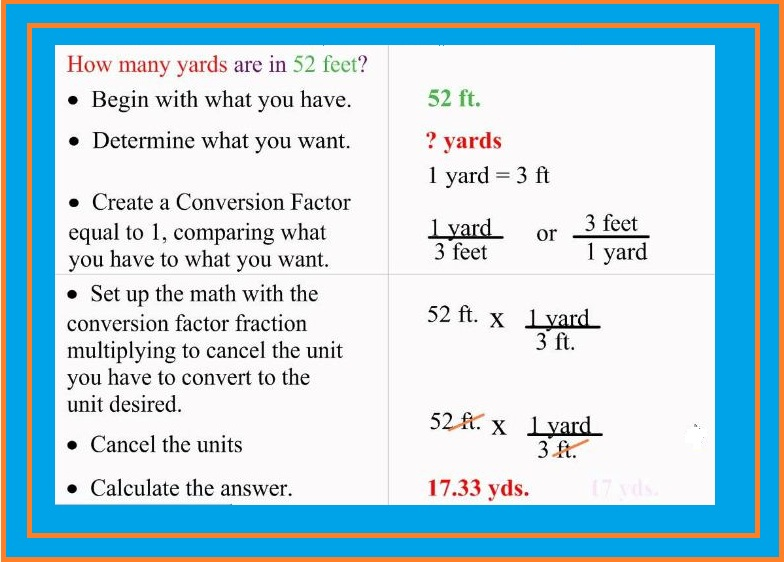### Law of conservation of massStatement : Matter can neither be created nor destroyed.

### Law of definite proportions### Law of multiple proportions

Statement : If two elements can combine to form more than one compound, the masses of one element that combine with a fixed mass of the other element, are in the ratio of small whole numbers. This law was given by Dalton in 1803.

e.g Hydrogen +oxygen → water

\ \ \ \ \ \ \ 2g \ \ \ \ \ \ \ 16g \ \ \ \ \ \ \18g

Hydrogen + Oxygen → Hydrogen peroxide

2g \ \ \ \ \ \ \ \ \ 32 g \ \ \ \ \ \ \ \ \ \ \ \ 34g

Here, the ratio of masses of oxygen (i.e. 16 g and 32 g) which combine with a fixed mass of hydrogen (2 g) is 1 : 2.

### Gay Lussac's law of gaseous volume

Statement : When gases combine or are produced in a chemical reaction they do so in a simple ratio by volume provided all gases are at
same temperature and pressure.

Hydrogen + Oxygen → Water

\ \ \ \ 100 \ mL \ \ \ \ \ \ \ 50 \ mL \ \ \ \ \ \ \100 \ mL

Here, ratio of volumes of hydrogen and oxygen is 2:1.

This law is also known as the law of definite proportions by volume.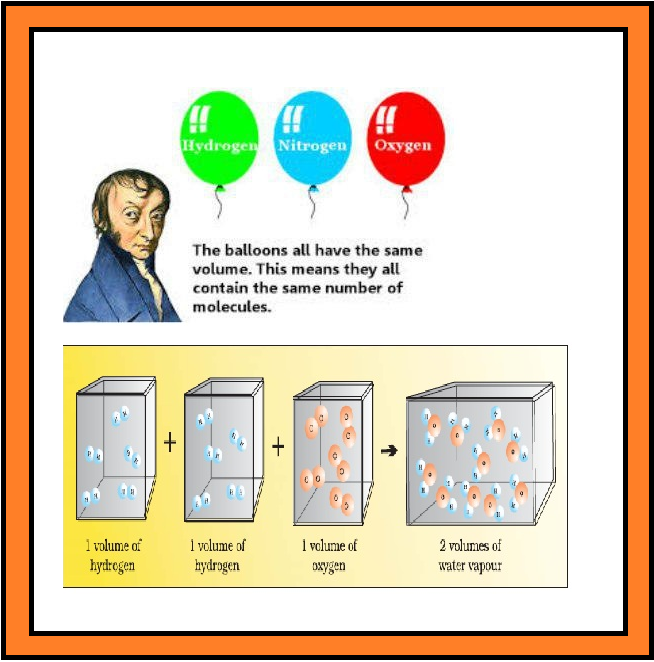Statement : Equal volumes of gases at the same temperature and pressure should contain equal number of molecules.

### Dalton's atomic theory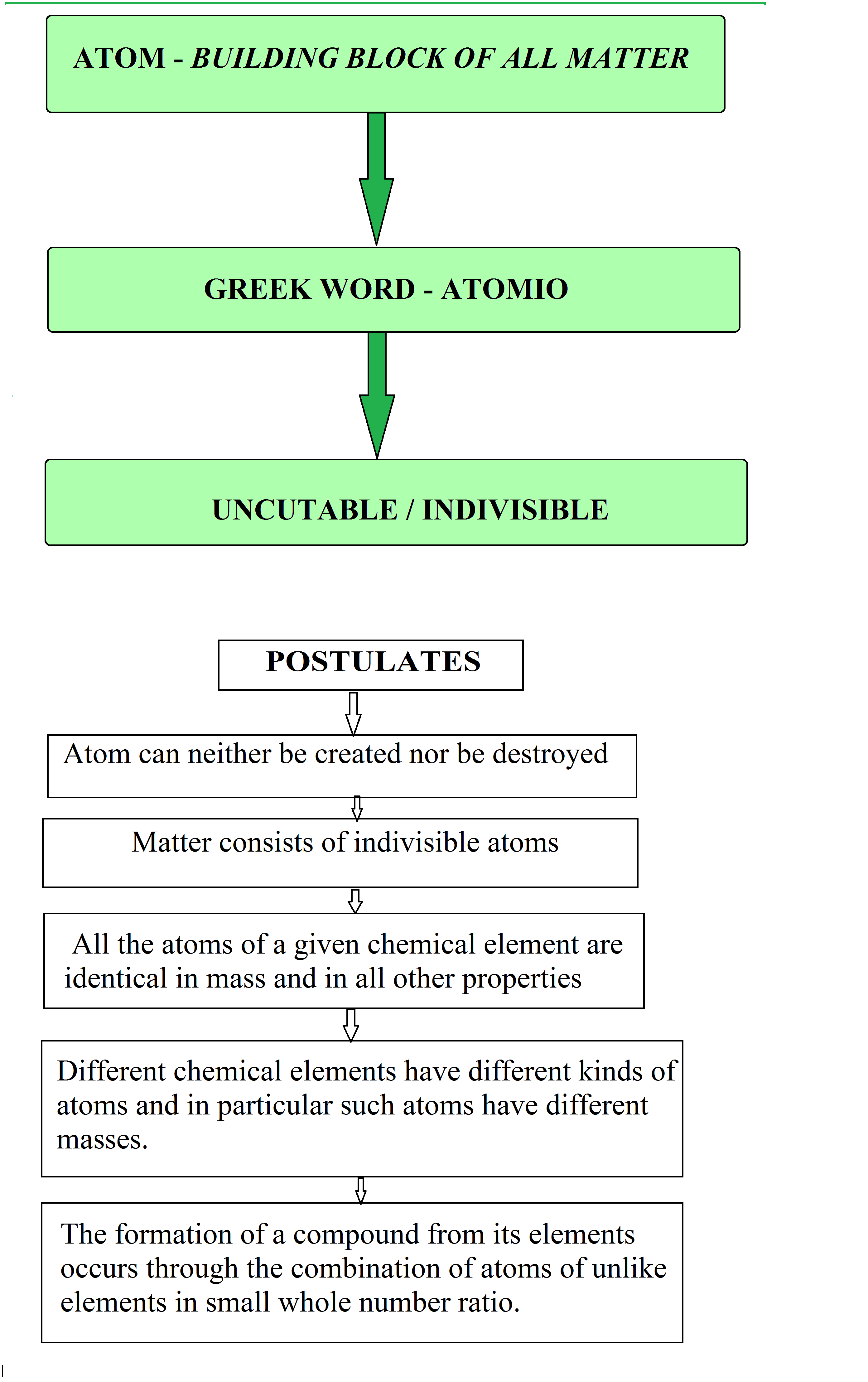### Mole concept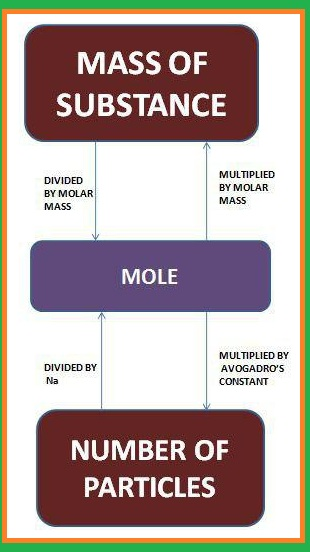### Molar mass### Percentage composition

Percentage composition gives the idea about the purity of a given sample by analyzing the given data.

Mass % of an element = text(mass of that element in the compound)/text(molar mass of the compound)

e.g. For H_2O

Molar mass of water = 18.02g

Mass % of H = (2xx1.008)/18.02 xx100

Mass % of O = 16.00/18.02 xx100= 88.79

### Empirical formula and molecular formula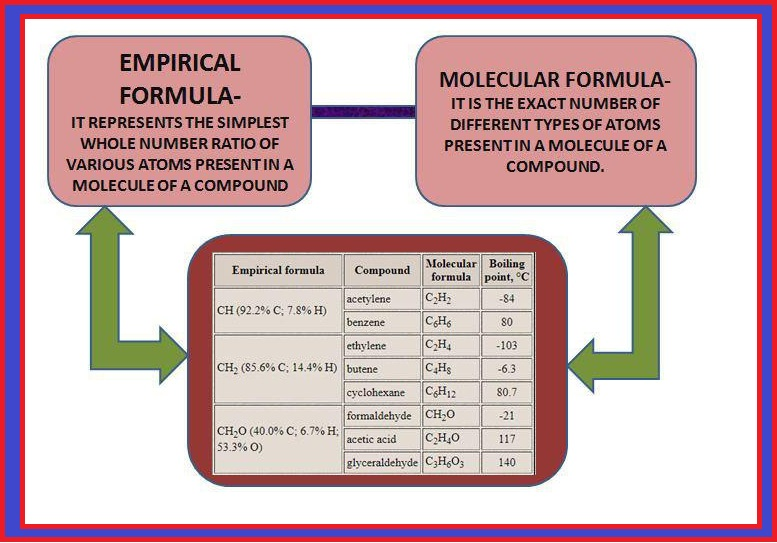### Limiting reagents### Concentration terms